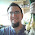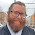## Wednesday, December 27, 2017

### Random Generators in ExcelAKA my day job
Today I'm taking a little detour away from in-game mechanics and lore to talk about a way to make your games easier to run. I've been using these tools for my recent games, and it's really saved me a lot of headache.

Most of the DMs I know write their games either in a text document or on paper. It usually consists of some worldbuilding notes, some shorthand monster stats, and some loot for the players. However, more and more people are using online services like r/BehindTheTables, donjon, or D&D Beyond to create reference materials and augment their games with a level of in-session randomness that adds excitement for the DM as well as the PCs.

Well, for those of us who play in our own worlds, use house rules, or even use our own RPGs, tools like that don't quite work. So, I started making my games in Microsoft Excel.

The benefits here are awesome: I can pull my shorthand statblocks, add any number of random tables, and even transition between scenes without tedious scrolling. In fact, my recent Campaign of Chaos game was completely within excel - no books, no paper, no text files!

Let's start with the basic excel functions we'll be using in this article. For the truly uninitiated, an excel function starts with an equals sign which is followed by a word (usually shortened for ease of use). So a good function to add up some values would be =SUM. But what values? Well, we need to add arguments, or parameters, for our function. So let's say we want to add up the values in column A, from A1 down to A50. The SUM formula can understand excel's table notation (A1:A50), so we just put that in parenthesis after the formula. If a formula has more than one argument, they can be separated by a comma. Entering =SUM(A1:A50) will give us the answer we want! And it's a lot easier than putting in =A1+A2+A3+....etc.

So here's the four most powerful formulas you can use to make random tables in Excel!
• RANDBETWEEN(
• This function generates a random number between any two limits you want
• The arguments for RANDBETWEEN are
• the lower limit of the random number set
• the upper limit of the random number set
• So, =RANDBETWEEN(1,20) will create a d20 roll
• Note that every time you change a cell, this value will recalculate, so it's best not to store vital information on a random generator
• VLOOKUP(
• The V stands for vertical
• This function looks at a table somewhere in excel, checks the first column (the vertical dimension of the table) for a specific value, and then outputs the value on that row which is in a specified column
• The arguments for VLOOKUP are
• what value you want to look up in the first column of the table
• the table itself
• which column's value you want to output from the row that contains the first value
• a TRUE/FALSE argument that specifies if the match in the first column needs to be exact. This usually isn't important unless you have a lot of similar items in your first column. FALSE means an exact match, so I usually put it there for good measure.
• So if you had the trinket table (PHB pg. 160) loaded into an excel spreadsheet in columns A and B, the formula =VLOOKUP(13,A1:B100,2,FALSE) would return "A tooth from an unknown beast"
• MATCH(
• This function looks at a list of values and finds a particular value. Then it outputs how many rows down that item is.
• The arguments for MATCH are
• what item we want to look up
• the list itself
• an optional argument that specifies if you want an exact match (0), the closest match equal to or less than your item (1), or the closest match equal to or greater than your item (-1)
• So let's use the Trinket list again (PHB pg. 160), loaded into columns A and B in a spreadsheet. The formula =MATCH("A tooth from an unknown beast",B1:B100,0) would return "13"
• CONCATENATE(
• This function takes two or more cell values and outputs them in the same cell.
• The argument for CONCATENATE is
• the text or cell we want to combine. You can have as many of these as you want, separated by a comma, of course.
• So if Cell A1 has "Dungeons" in it, and cell A2 has "Dragons" in it, the formula =CONCATENATE(A1," & ",A2) would return "Dungeons & Dragons". Notice that I had to include spaces around the & symbol. Concatenate doesn't understand you are inputting different words!

So let's try a basic table. Your players just found a Potion of Resistance. Good for them! But, the Barbarian asks, what kind of Potion of Resistance? What sort of damage does it resist?

Well, first off, we need to make a table with the different kinds of resistances.
Perfect! Now, we'll need three of our four formulas, depending on how fancy we want this to get.

At the most basic level, we can add the formula =RANDBETWEEN(1,10). That will give us a random number between 1 and 10, then we can just look up the value. It's Cold damage, you say. Your players are appeased.

But what if you want the sheet to spit out the answer for you? This could be the case if you don't want your sheet crowded up with tables. You don't have time to look it up yourself! And with bigger tables, that can definitely be the case.

The easy way is that you can look up the value from your random number generator. Let's say you put the RANDBETWEEN formula in cell C1. Then, your lookup formula would be =VLOOKUP(C1,A1:B10,2,FALSE). Now, the VLOOKUP cell will say "Cold", because it's taking the 2 from RANDBETWEEN and using it to look up the proper word!

The trickier way is using nestled formulas. Since VLOOKUP is just looking at RANDBETWEEN for its value, you can simply insert the RANDBETWEEN formula right into VLOOKUP! Your formula would then be =VLOOKUP(RANDBETWEEN(1,10),A1:B10,2,FALSE). Though it's more complex, it'd get you the exact same answer!

But let's take it one step further. Suppose you want the players to be able to look at this sheet. So it can't just say "Cold". Well, that's where our CONCATENATE formula comes into play.

If you have your VLOOKUP formula in cell C1, you can create a formula that reads: =CONCATENATE("Potion of ",C1," Resistance"). Then, that cell will read "Potion of Cold Resistance", or whatever value your random number generator has produced. Of course, if you want, you could nestle the VLOOKUP into the CONCATENATE formula as well, but I'll leave that up to you.

If you're not following, I'd highly suggest opening up excel and trying to replicate this sheet as you see above. Doing is the best way to learn!

Now, let's try something a little more complex. I'm going to use the MATCH formula to make a sheet that will tell a new player which damage die to roll based on which weapon they want their rogue to attack with.

We start with our input format. I like to highlight the boxes that require input. Note that right now this is nothing but text - no formulas at all.
Next we'll need a list of the weapons the rogue has.
Assign each one a number, and add the damage die it uses.
Now we have a VLOOKUP table! But we aren't quite ready for that yet. First, we need to use a MATCH formula to get the row number of the player's input. Notice I'm using the optional argument to make sure we get an exact match. It isn't necessary, but it's good practice for when you move to more complex tables.
As you can see, it returns the value 1, because "Dagger" is in the first row. Now, we can use that 1 value to create a VLOOKUP.
Perfect!

This can go even deeper, of course. Once you learn the basic building blocks of the system, you can make pretty much anything.

Here's the sheet I used for that Campaign of Chaos game I mentioned above. The players were exploring a city, so I had the city map and a description of the area they were in that popped up when I put in a reference number (thanks, VLOOKUP!). I also had four randomly generated NPCs (based on the tables found in the DMG pg. 89) for them to interact with/kill.

But the coolest part was the Quests. I had three mini-quests laid out for each area, and the number in the "Quests Completed" column modified a VLOOKUP table so that I always got the next quest in the series. I even had the endgame written up once the total number of quests completed was 15 or more. This required another formula, the IF( formula, but if you're curious about that one I'd suggest you look it up yourself!

Here's a sheet that rolls on the random encounter table for Maze of the Blue Medusa, then gives me the 5th edition statblock for the monster I rolled! Again, this is all using RANDBETWEEN( and VLOOKUP(. I even used a MATCH( formula so I could type in the zone the PCs were exploring in cell B3. Easy as pie!

Finally, in case you don't have an up-to-date version of excel, everything I've shown here can be replicated in Google Sheets, which is free!
Here's a downtime generator that I've made based on the tables from Xanathar's Guide to Everything (XGE pg. 125). The box at the bottom uses a match formula to find what downtime activity you want to do.
Then, once you type in an activity, this dialogue shows up. I highlighted the cells that I wrote my information in, and the output of that information. I actually had to recreate the random magic item generators in the DMG to make this work - it took quite a while! Here's a link to the spreadsheet itself. You won't be able to modify it in-browser, but feel free to download it and try it out! If you happen to find a bug, feel free to comment here or in the sheet and I can update it.

I hope this was exciting or at least enlightening for you. With advancements in technology, in-game randomness doesn't have to involve the DM sitting behind the screen rolling dice for 5 minutes. You can have your procedurally-generated cake and eat it, too!

1.1.